# 有源低通滤波器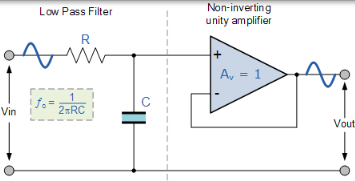## 有源低通滤波器

### 一阶低通滤波器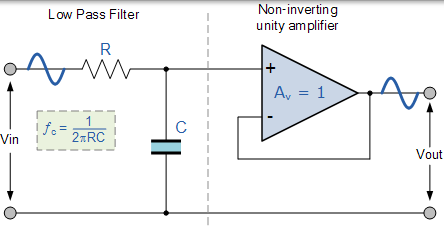### 具有放大功能的有源低通滤波器$$\mathrm { DC } \text { gain } = \left( 1 + \frac { \mathrm { R } _ { 2 } } { \mathrm { R } _ { 1 } } \right)$$

### 一阶低通滤波器的增益

$$\text { Voltage Gain, } ( A v ) = \frac { \text {Vout} } { V i n } = \frac { A _ { F } } { \sqrt { 1 + \left( \frac { f } { f c } \right) ^ { 2 } } }$$

• AF =滤波器的通带增益（ 1 + R2 / R1 ）
• ƒ = 输入信号的频率，单位为赫兹（Hz）
• ƒc =以赫兹为单位的截止频率（Hz）

1. 在非常低的频率下，ƒ<ƒc 时， $\frac { \mathrm { Vout } } { \mathrm { Vin } } \cong \mathrm { A } _ { \mathrm { F } }$
2. 在截止频率，ƒ=ƒc 时， $\frac { Vout } { \operatorname { Vin } } = \frac { A _ { F } } { \sqrt { 2 } } = 0.707 \mathrm { A } _ { \mathrm { F } }$
3. 在非常高的频率下，ƒ>ƒc 时， $\frac { \text { Vout } } { \text { Vin } } < A _ { F }$

### 电压增益幅度（dB）

$$\begin{array} { c } { \mathrm { AV } ( \mathrm { dB } ) = 20 \log _ { 10 } \left( \frac { \mathrm { Vout } } { \mathrm { Vin } } \right) } \\ { \therefore - 3 \mathrm { dB } = 20 \log _ { 10 } \left( 0.707 \frac { \mathrm { Vout } } { \mathrm { Vin } } \right) } \end{array}$$

## 有源低通滤波器示例 No1

$$A _ { F } = 1 + \frac { R _ { 2 } } { R _ { 1 } } = 10$$

$$R _ { 2 } = ( 10 - 1 ) x R _ { 1 } = 9 x | k \Omega = 9 k \Omega$$

$$\text {Gain in } d B = 20 \log A = 20 \log 10 = 20 d B$$

$$f _ { C } = \frac { 1 } { 2 \pi R C } H z$$

$$C = \frac { 1 } { 2 \pi f c R } = \frac { 1 } { 2 \pi x 159 \times 10 k \Omega } = 100 n F$$

### 低通滤波器电路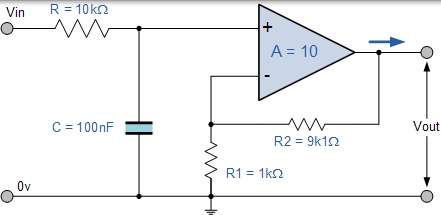### 频率响应曲线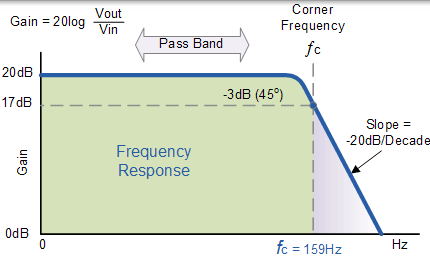$$f _ { C } = \frac { 1 } { 2 \pi C R _ { 2 } } \text { Hertz }$$

### 简化的非反相放大器滤波电路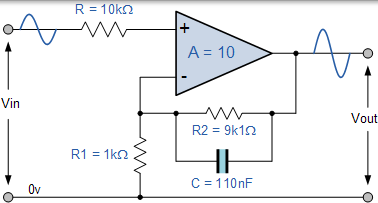### 等效反相放大器滤波电路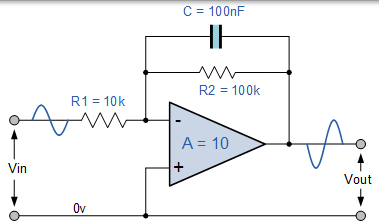## 二阶低通有源滤波器

### 二阶有源低通滤波器电路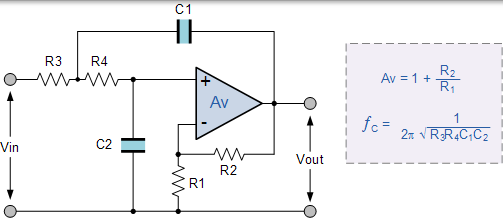### 级联电压增益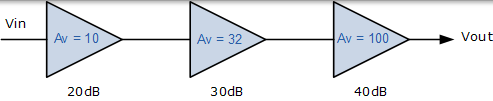\begin{aligned} \mathrm { Av } & = \mathrm { Av } _ { 1 } \times \mathrm { Av } _ { 2 } \times \mathrm { AV } _ { 3 } \\ \mathrm { Av } ( \mathrm { dB } ) & = 20 \log _ { 10 } ( 32,000 ) \\ \mathrm { Av } ( \mathrm { dB } ) & = 90 \mathrm { d } \mathrm { B } \\ \mathrm { Av } ( \mathrm { dB } ) & = 90 d B \\ 90 \mathrm { d } \mathrm { B } & = 20 \mathrm { d } \mathrm { B } + 30 \mathrm { d } \mathrm { B } + 40 \mathrm { d } \mathrm { B } \end{aligned}